Скачать презентацию Multicommodity Rent or Buy Approximation Via Cost Sharing

• Количество слайдов: 41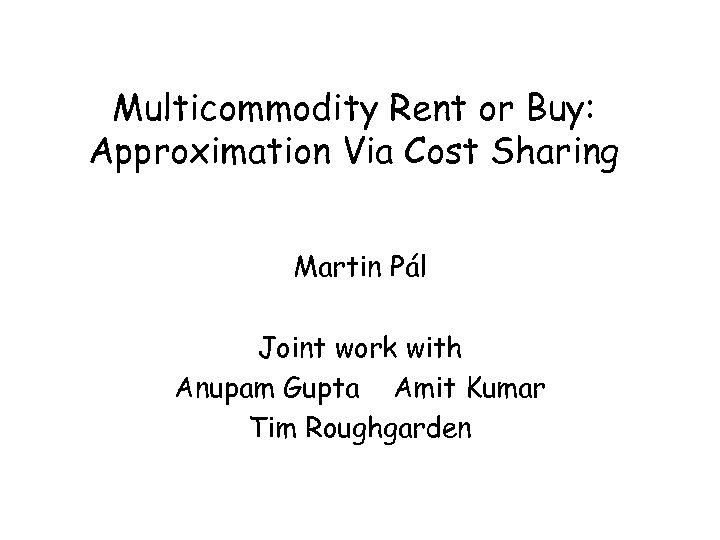Multicommodity Rent or Buy: Approximation Via Cost Sharing Martin Pál Joint work with Anupam Gupta Amit Kumar Tim Roughgarden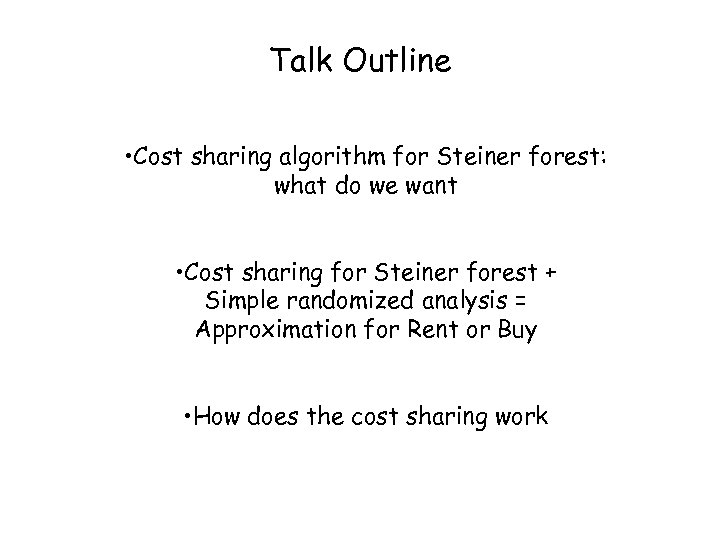Talk Outline • Cost sharing algorithm for Steiner forest: what do we want • Cost sharing for Steiner forest + Simple randomized analysis = Approximation for Rent or Buy • How does the cost sharing work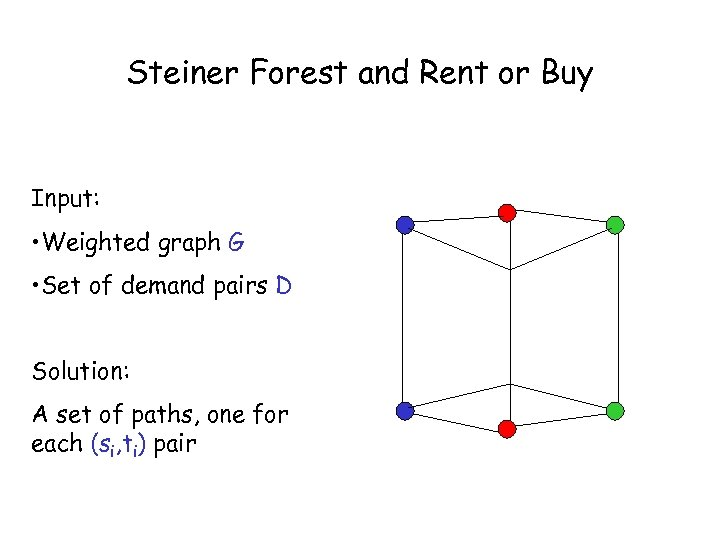Steiner Forest and Rent or Buy Input: • Weighted graph G • Set of demand pairs D Solution: A set of paths, one for each (si, ti) pair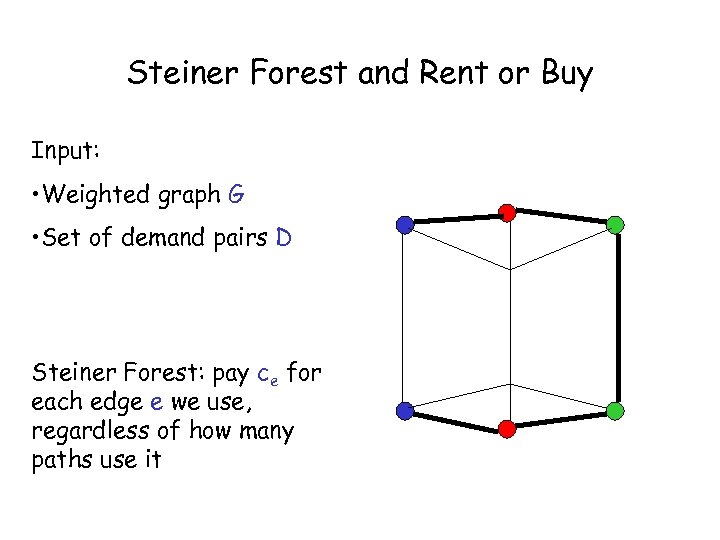Steiner Forest and Rent or Buy Input: • Weighted graph G • Set of demand pairs D Steiner Forest: pay ce for each edge e we use, regardless of how many paths use it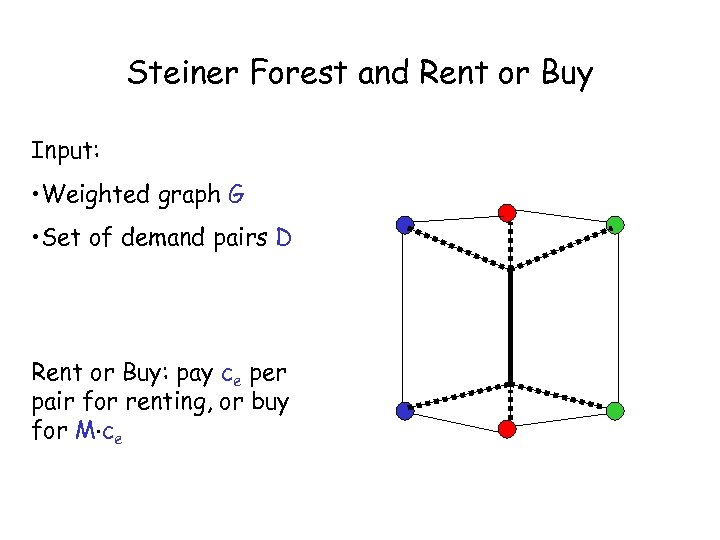Steiner Forest and Rent or Buy Input: • Weighted graph G • Set of demand pairs D Rent or Buy: pay ce per pair for renting, or buy for M ce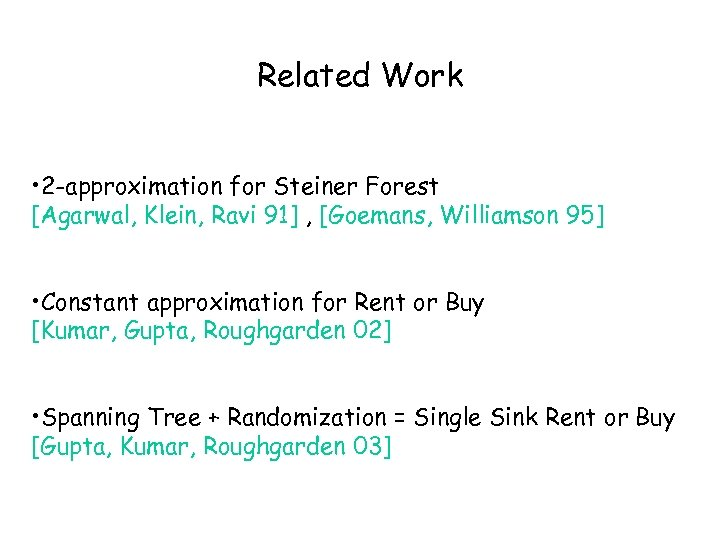Related Work • 2 -approximation for Steiner Forest [Agarwal, Klein, Ravi 91] , [Goemans, Williamson 95] • Constant approximation for Rent or Buy [Kumar, Gupta, Roughgarden 02] • Spanning Tree + Randomization = Single Sink Rent or Buy [Gupta, Kumar, Roughgarden 03]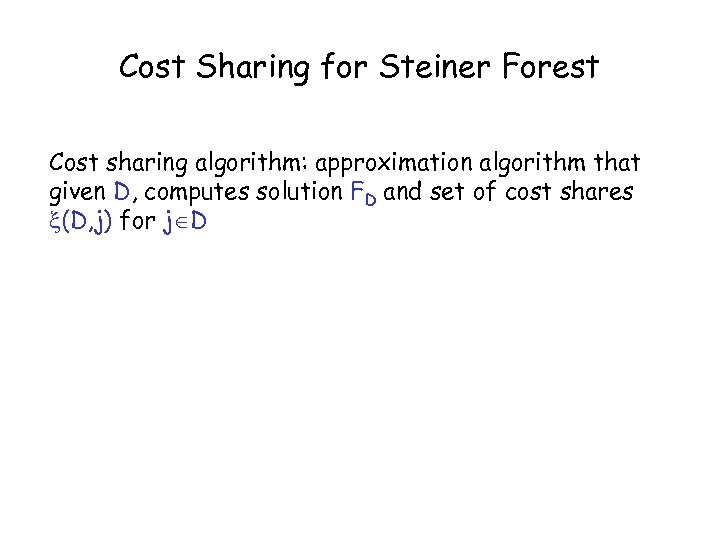Cost Sharing for Steiner Forest Cost sharing algorithm: approximation algorithm that given D, computes solution FD and set of cost shares (D, j) for j D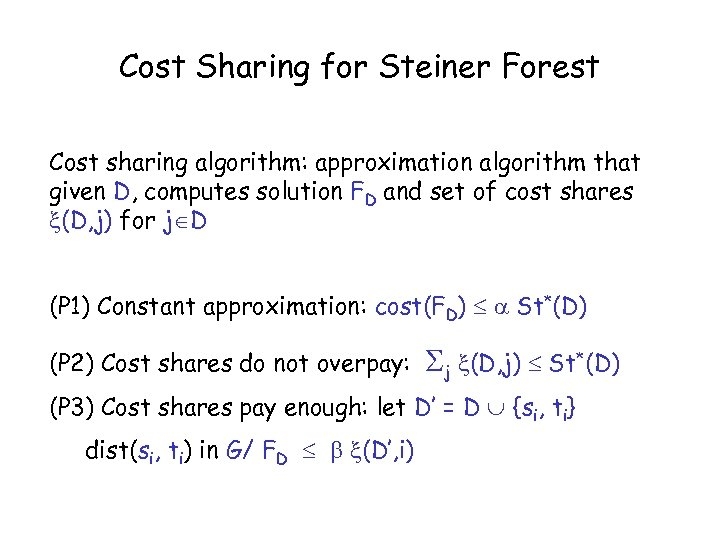Cost Sharing for Steiner Forest Cost sharing algorithm: approximation algorithm that given D, computes solution FD and set of cost shares (D, j) for j D (P 1) Constant approximation: cost(FD) St*(D) (P 2) Cost shares do not overpay: j (D, j) St*(D) (P 3) Cost shares pay enough: let D’ = D {si, ti} dist(si, ti) in G/ FD (D’, i)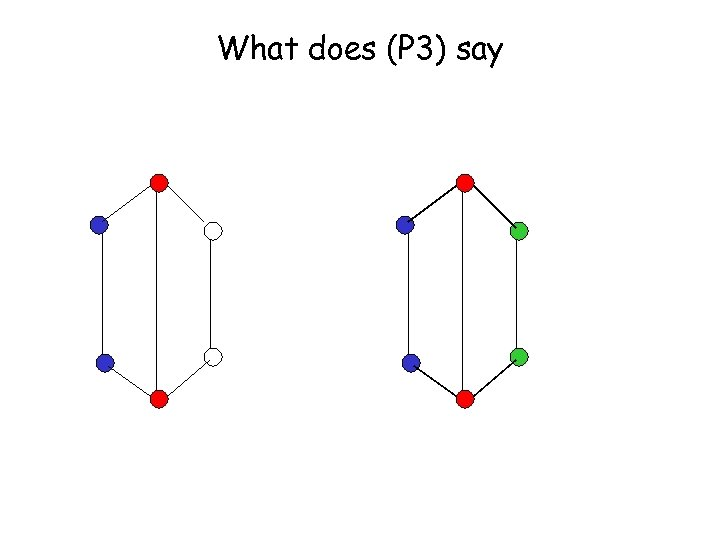What does (P 3) say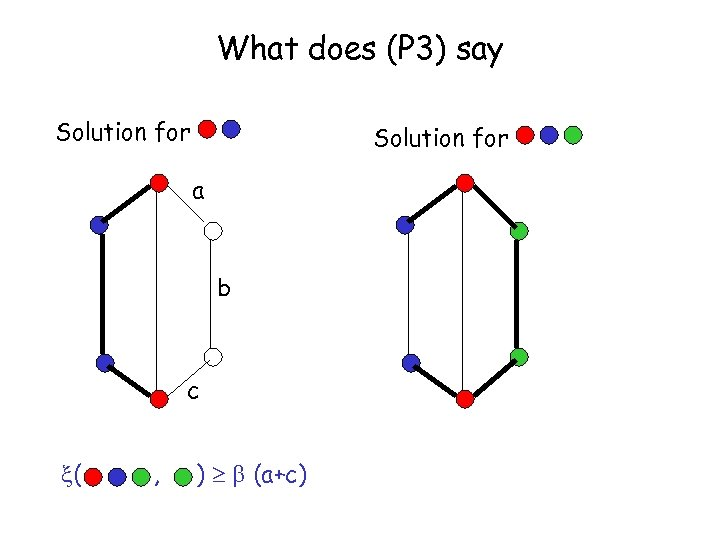What does (P 3) say Solution for a b c ( , ) (a+c)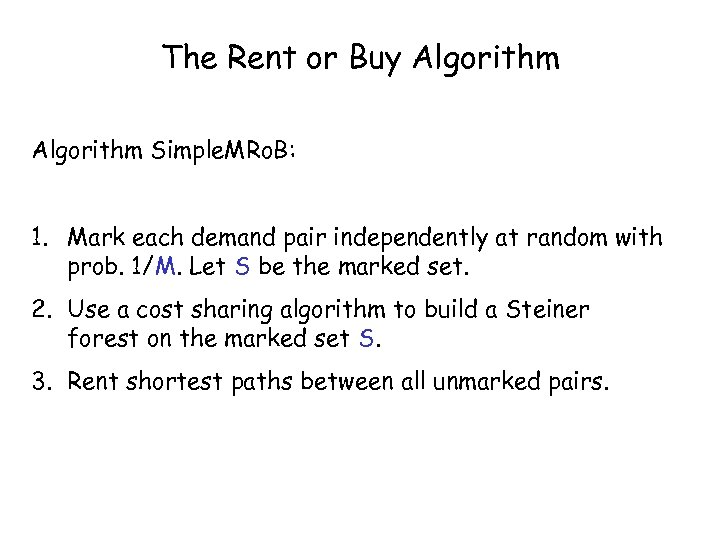The Rent or Buy Algorithm Simple. MRo. B: 1. Mark each demand pair independently at random with prob. 1/M. Let S be the marked set. 2. Use a cost sharing algorithm to build a Steiner forest on the marked set S. 3. Rent shortest paths between all unmarked pairs.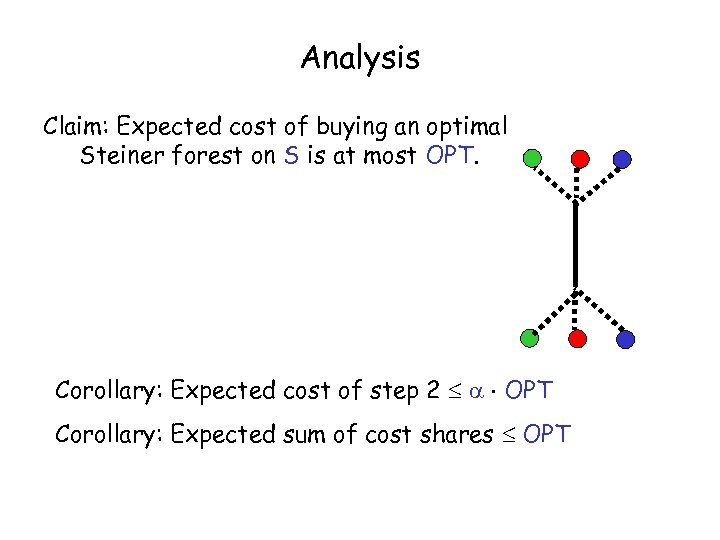Analysis Claim: Expected cost of buying an optimal Steiner forest on S is at most OPT. Corollary: Expected cost of step 2 OPT Corollary: Expected sum of cost shares OPT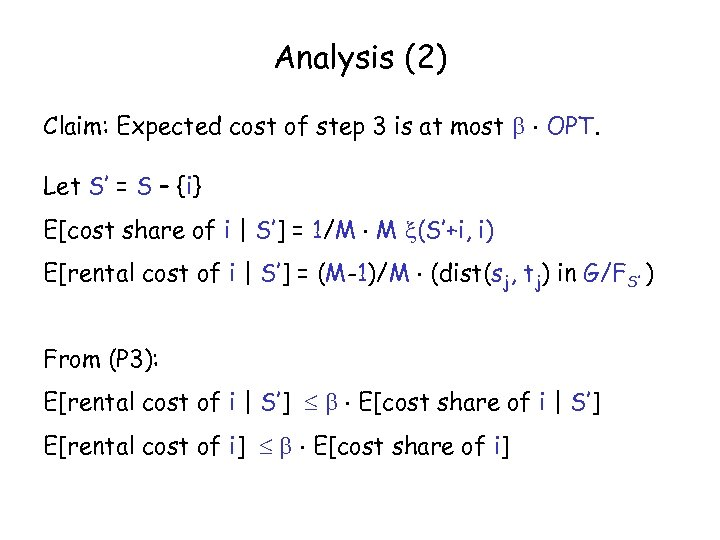Analysis (2) Claim: Expected cost of step 3 is at most OPT. Let S’ = S – {i} E[cost share of i | S’] = 1/M M (S’+i, i) E[rental cost of i | S’] = (M-1)/M (dist(sj, tj) in G/FS’ ) From (P 3): E[rental cost of i | S’] E[cost share of i | S’] E[rental cost of i] E[cost share of i]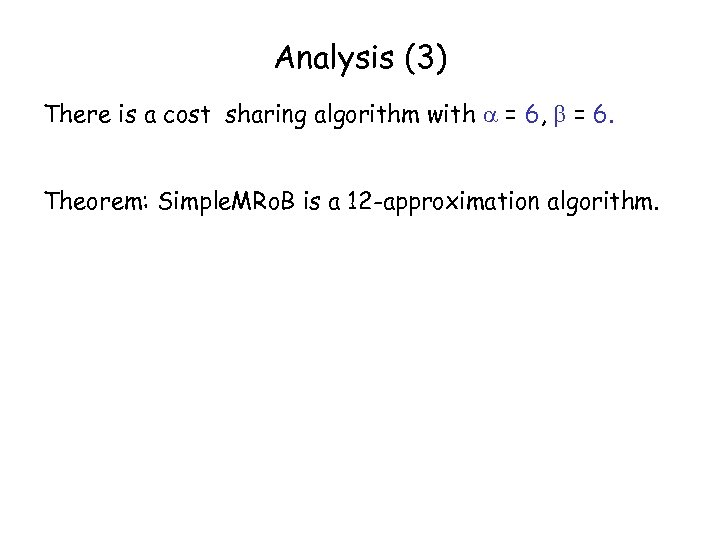Analysis (3) There is a cost sharing algorithm with = 6, = 6. Theorem: Simple. MRo. B is a 12 -approximation algorithm.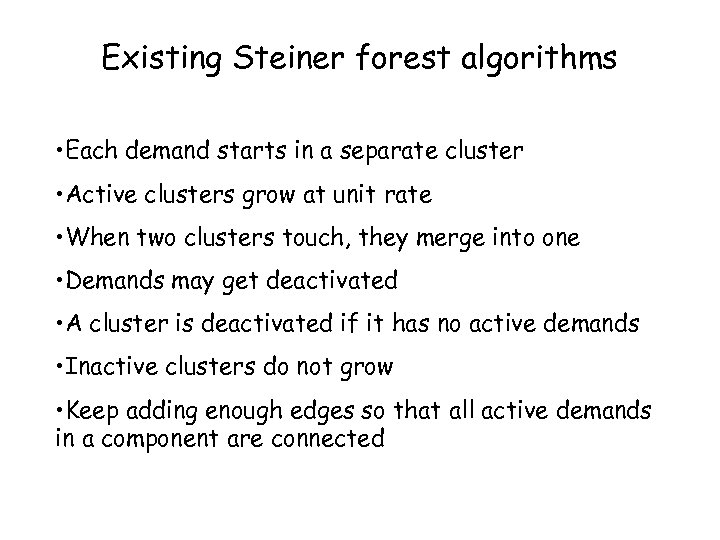Existing Steiner forest algorithms • Each demand starts in a separate cluster • Active clusters grow at unit rate • When two clusters touch, they merge into one • Demands may get deactivated • A cluster is deactivated if it has no active demands • Inactive clusters do not grow • Keep adding enough edges so that all active demands in a component are connected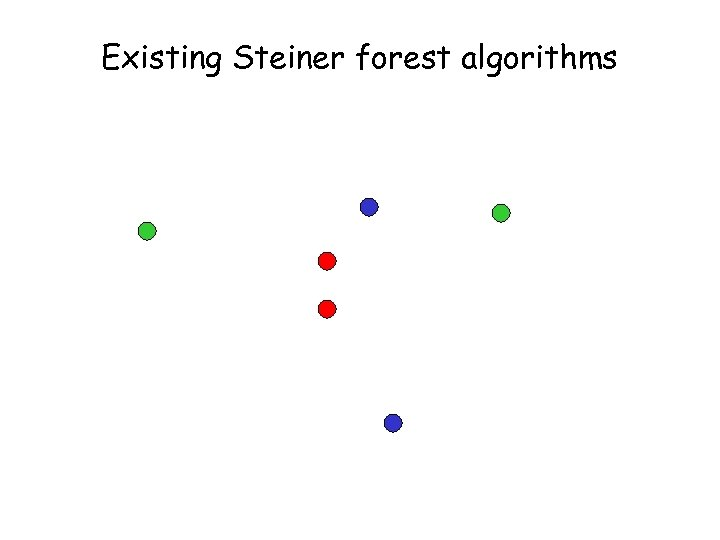Existing Steiner forest algorithms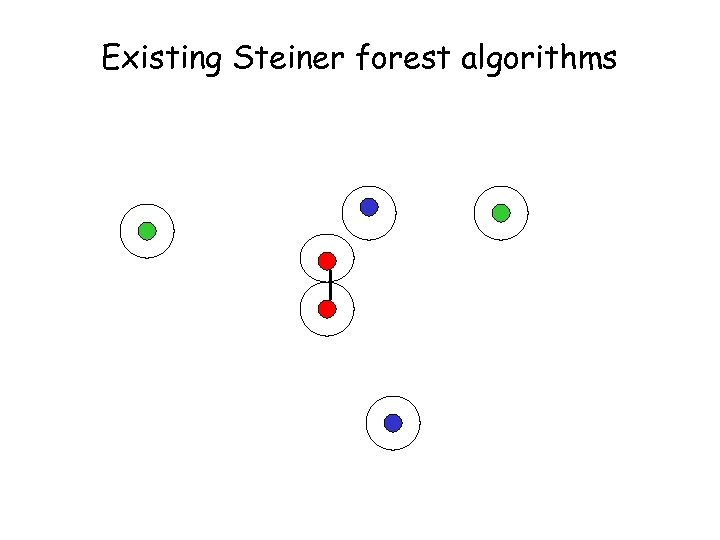Existing Steiner forest algorithms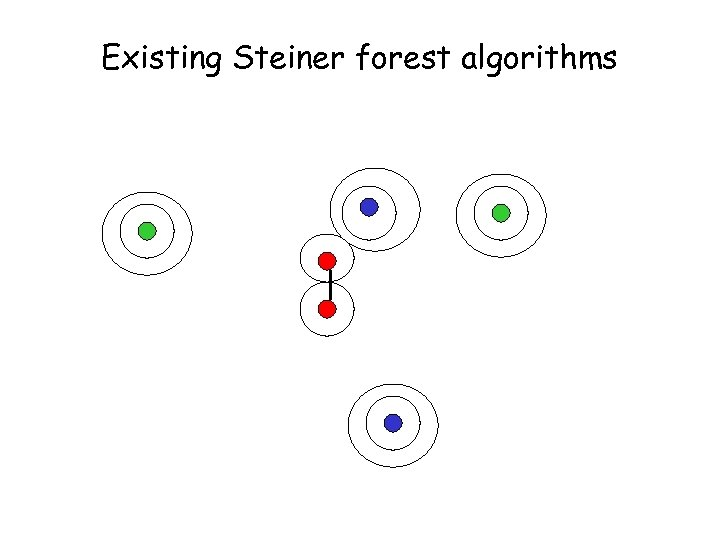Existing Steiner forest algorithms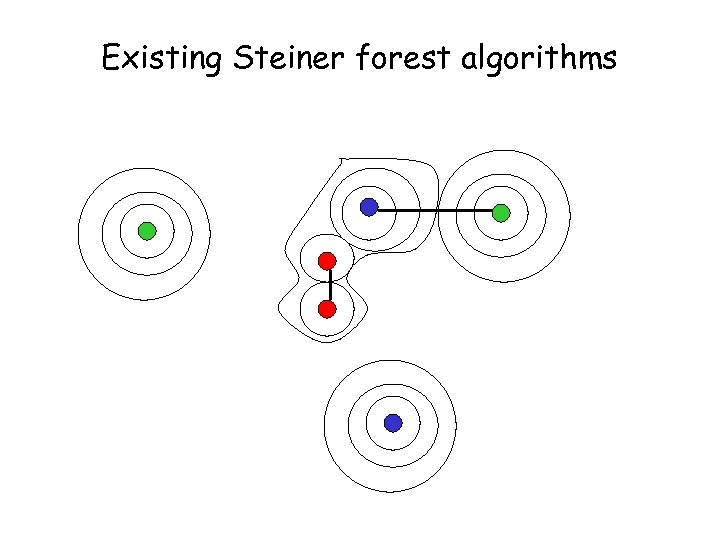Existing Steiner forest algorithms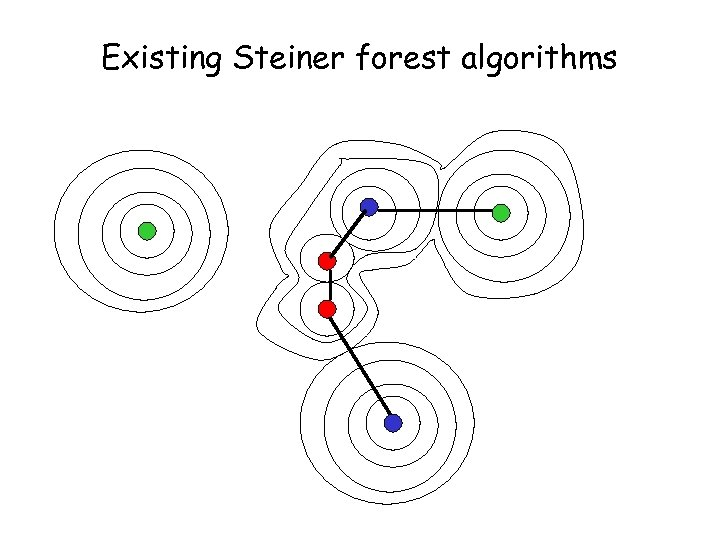Existing Steiner forest algorithms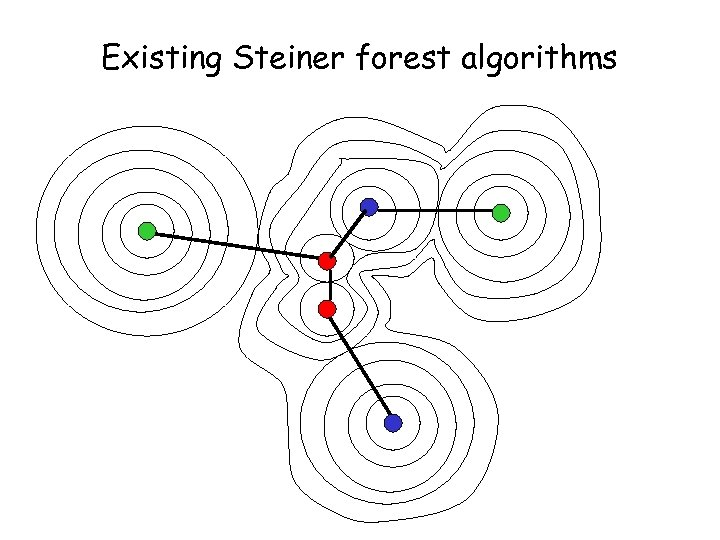Existing Steiner forest algorithms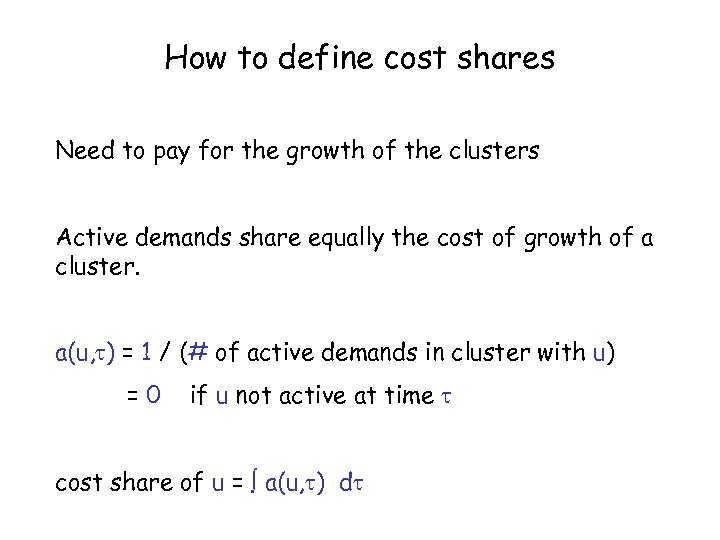How to define cost shares Need to pay for the growth of the clusters Active demands share equally the cost of growth of a cluster. a(u, ) = 1 / (# of active demands in cluster with u) =0 if u not active at time cost share of u = a(u, ) d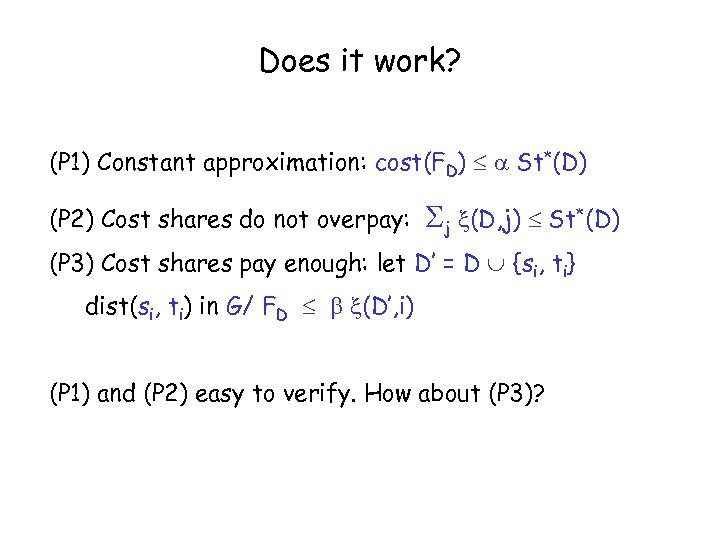Does it work? (P 1) Constant approximation: cost(FD) St*(D) (P 2) Cost shares do not overpay: j (D, j) St*(D) (P 3) Cost shares pay enough: let D’ = D {si, ti} dist(si, ti) in G/ FD (D’, i) (P 1) and (P 2) easy to verify. How about (P 3)?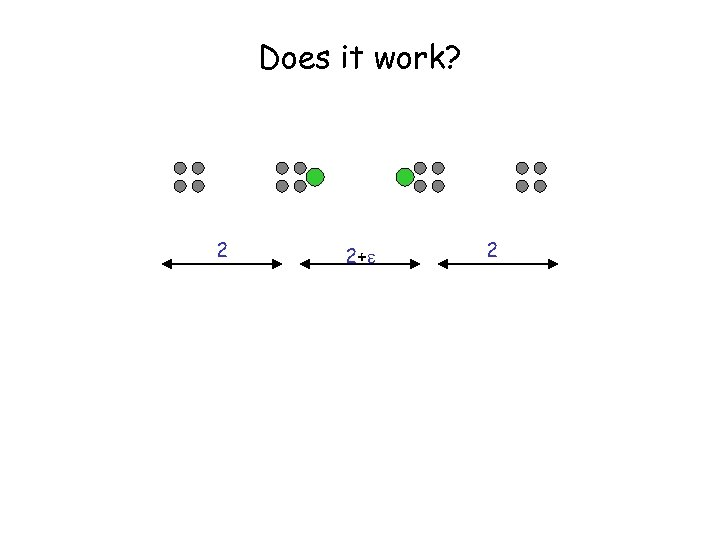Does it work? 2 2+ 2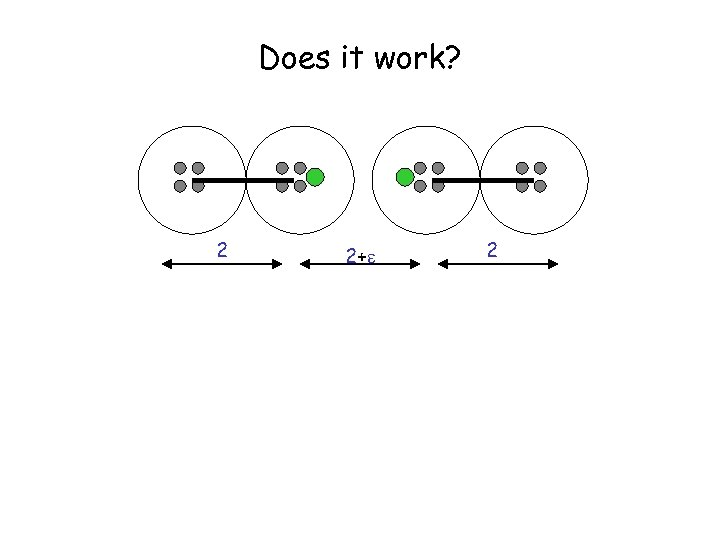Does it work? 2 2+ 2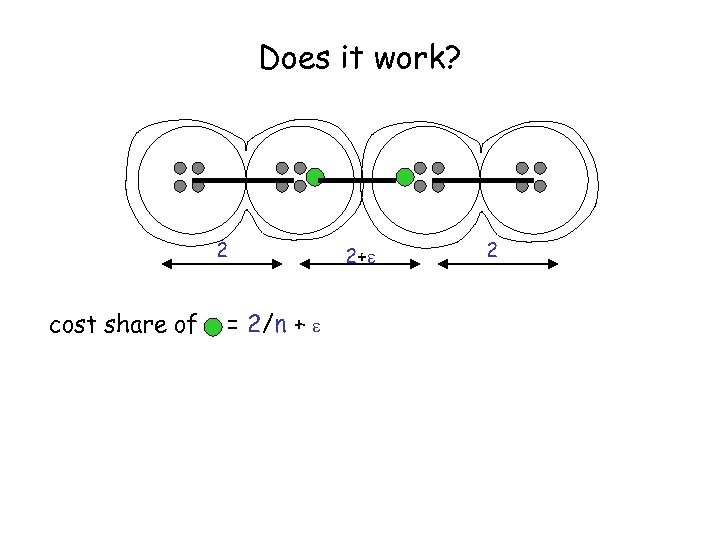Does it work? 2 cost share of = 2/n + 2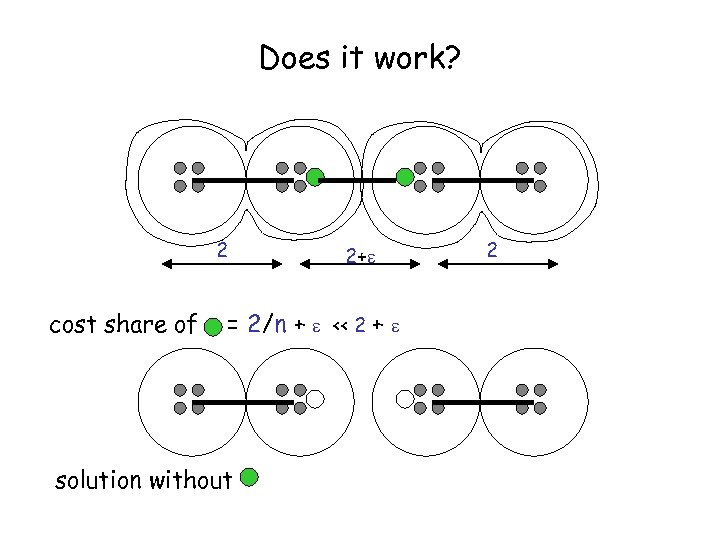Does it work? 2 cost share of 2+ = 2/n + << 2 + solution without 2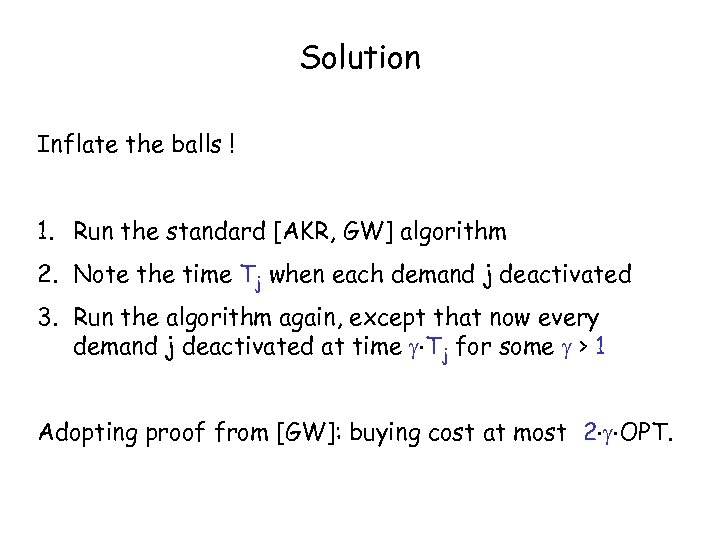Solution Inflate the balls ! 1. Run the standard [AKR, GW] algorithm 2. Note the time Tj when each demand j deactivated 3. Run the algorithm again, except that now every demand j deactivated at time Tj for some > 1 Adopting proof from [GW]: buying cost at most 2 OPT.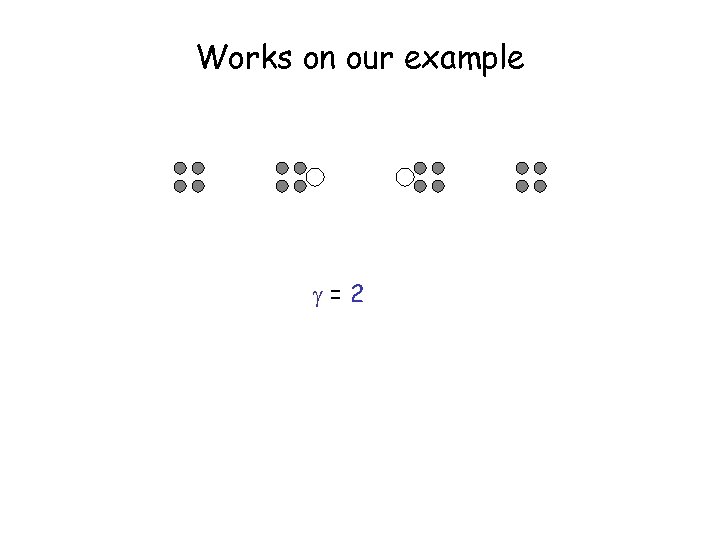Works on our example =2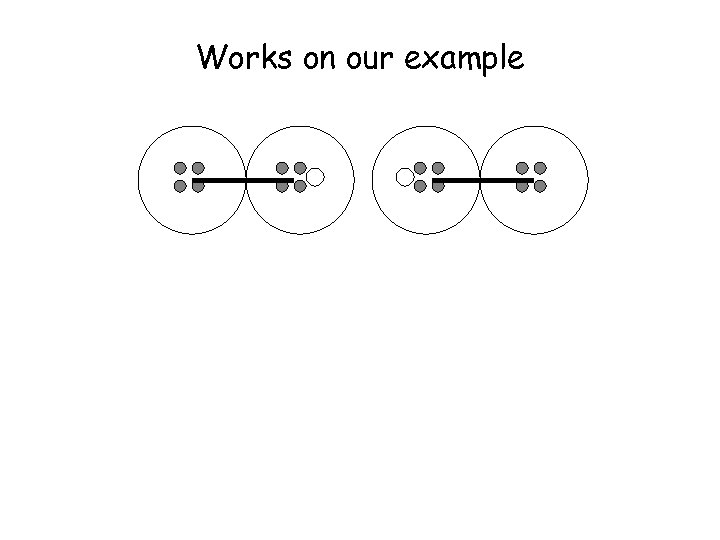Works on our example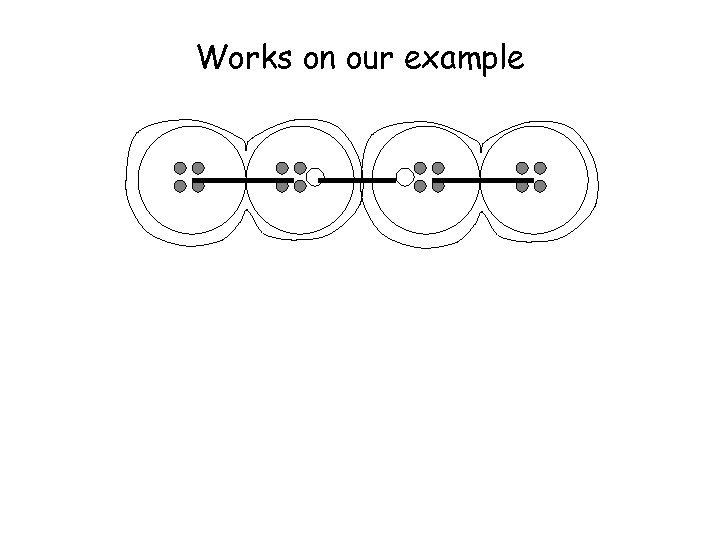Works on our example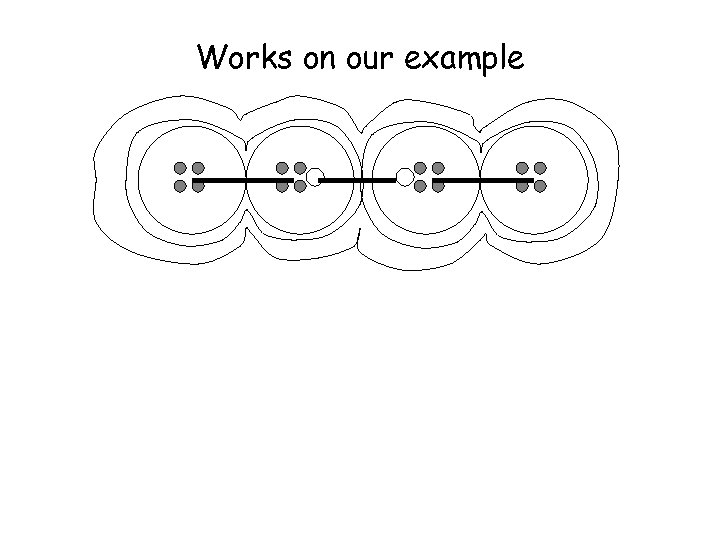Works on our example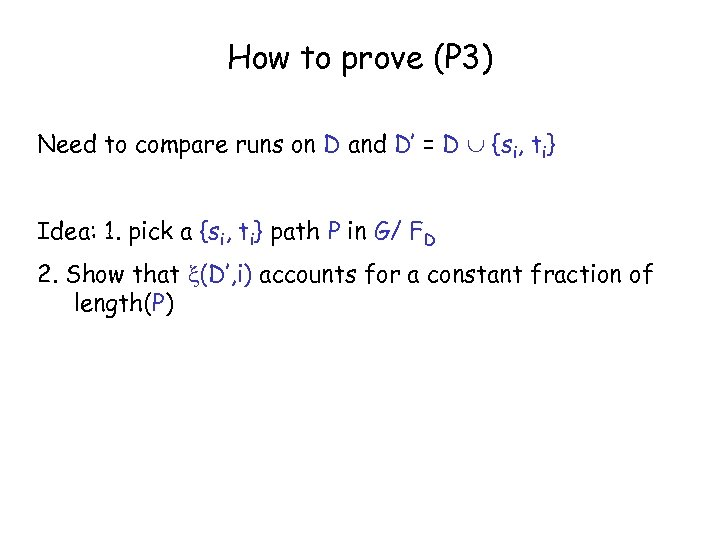How to prove (P 3) Need to compare runs on D and D’ = D {si, ti} Idea: 1. pick a {si, ti} path P in G/ FD 2. Show that (D’, i) accounts for a constant fraction of length(P)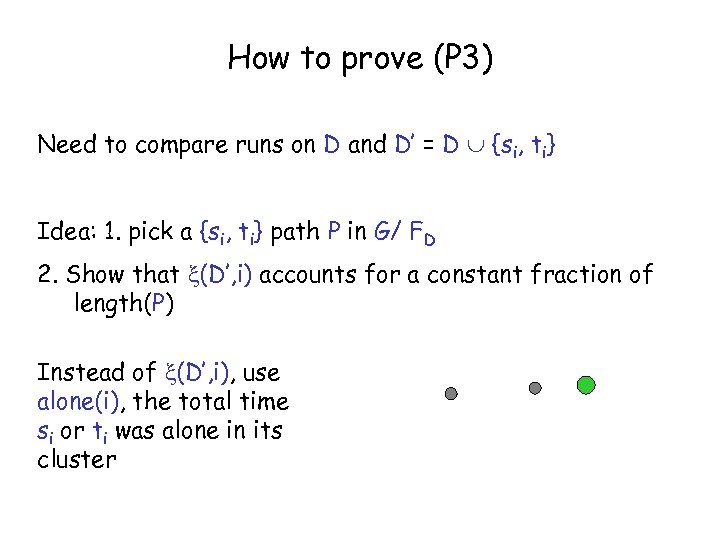How to prove (P 3) Need to compare runs on D and D’ = D {si, ti} Idea: 1. pick a {si, ti} path P in G/ FD 2. Show that (D’, i) accounts for a constant fraction of length(P) Instead of (D’, i), use alone(i), the total time si or ti was alone in its cluster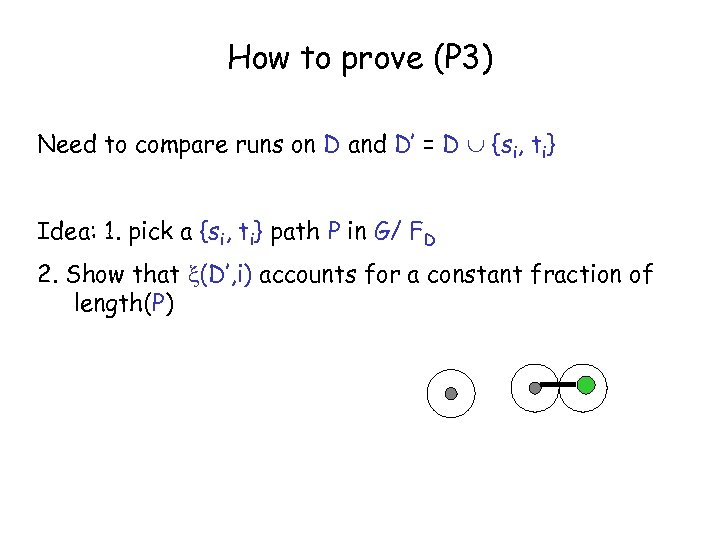How to prove (P 3) Need to compare runs on D and D’ = D {si, ti} Idea: 1. pick a {si, ti} path P in G/ FD 2. Show that (D’, i) accounts for a constant fraction of length(P)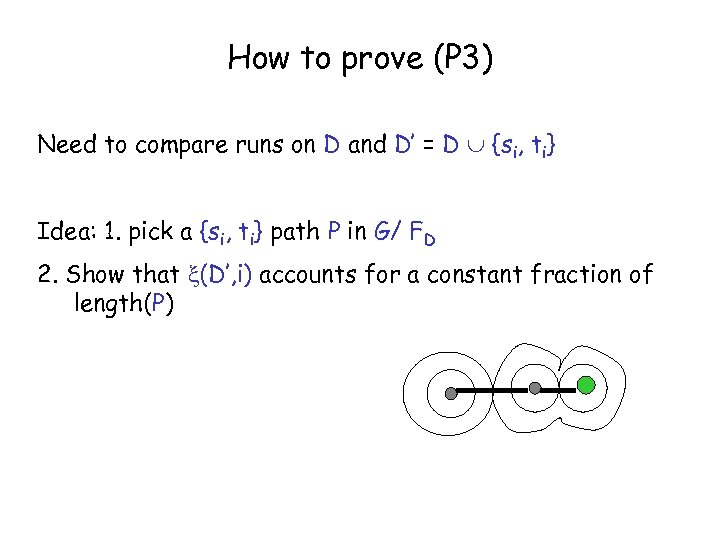How to prove (P 3) Need to compare runs on D and D’ = D {si, ti} Idea: 1. pick a {si, ti} path P in G/ FD 2. Show that (D’, i) accounts for a constant fraction of length(P)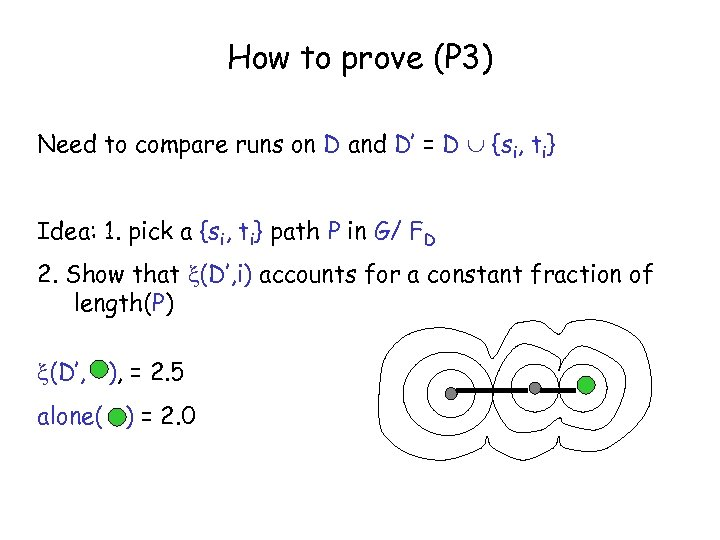How to prove (P 3) Need to compare runs on D and D’ = D {si, ti} Idea: 1. pick a {si, ti} path P in G/ FD 2. Show that (D’, i) accounts for a constant fraction of length(P) (D’, ), = 2. 5 alone( ) = 2. 0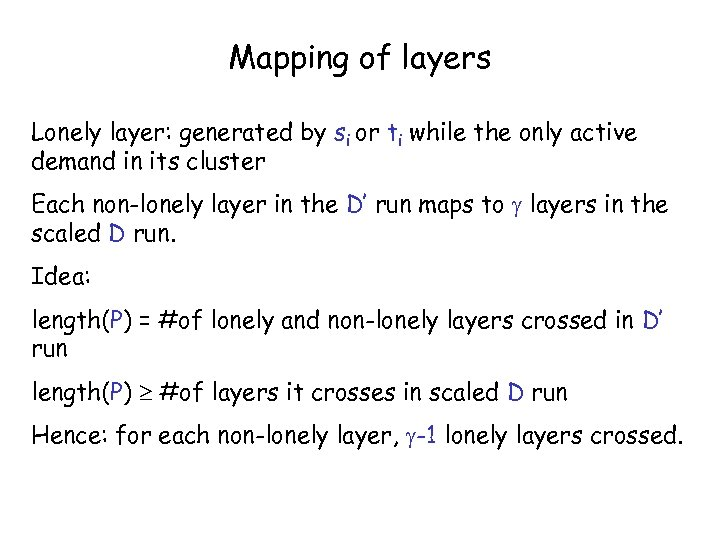Mapping of layers Lonely layer: generated by si or ti while the only active demand in its cluster Each non-lonely layer in the D’ run maps to layers in the scaled D run. Idea: length(P) = #of lonely and non-lonely layers crossed in D’ run length(P) #of layers it crosses in scaled D run Hence: for each non-lonely layer, -1 lonely layers crossed.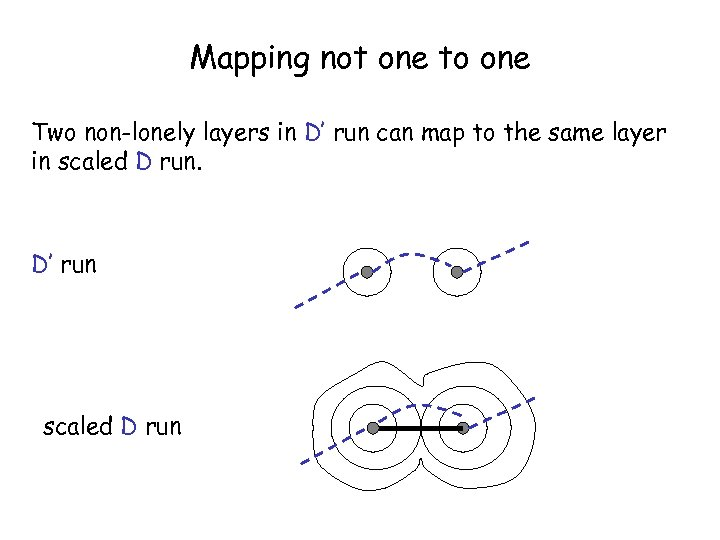Mapping not one to one Two non-lonely layers in D’ run can map to the same layer in scaled D run. D’ run scaled D run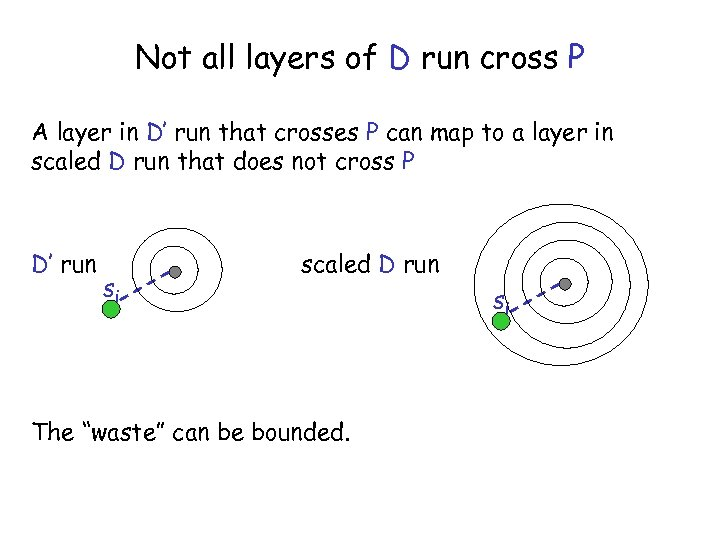Not all layers of D run cross P A layer in D’ run that crosses P can map to a layer in scaled D run that does not cross P D’ run si scaled D run The “waste” can be bounded. si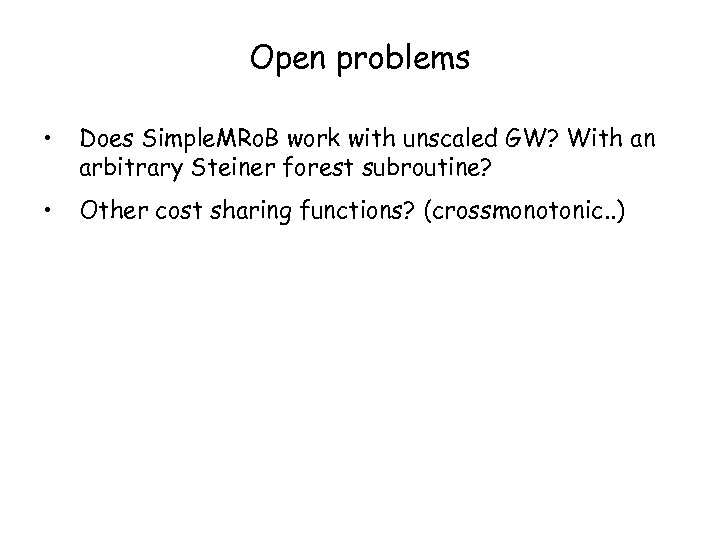Open problems • Does Simple. MRo. B work with unscaled GW? With an arbitrary Steiner forest subroutine? • Other cost sharing functions? (crossmonotonic. . )